Courses

# Forecasting Routing & Scheduling - MCQ Test 1

## 20 Questions MCQ Test GATE Mechanical (ME) 2022 Mock Test Series | Forecasting Routing & Scheduling - MCQ Test 1

Description
This mock test of Forecasting Routing & Scheduling - MCQ Test 1 for Mechanical Engineering helps you for every Mechanical Engineering entrance exam. This contains 20 Multiple Choice Questions for Mechanical Engineering Forecasting Routing & Scheduling - MCQ Test 1 (mcq) to study with solutions a complete question bank. The solved questions answers in this Forecasting Routing & Scheduling - MCQ Test 1 quiz give you a good mix of easy questions and tough questions. Mechanical Engineering students definitely take this Forecasting Routing & Scheduling - MCQ Test 1 exercise for a better result in the exam. You can find other Forecasting Routing & Scheduling - MCQ Test 1 extra questions, long questions & short questions for Mechanical Engineering on EduRev as well by searching above.
QUESTION: 1

### Which one of the following forecasting techniques is not suited for making forecasts for planning production schedules in the short range?

Solution:

Moving, average, Exponential moving average is used for short range.
Regression is used for short and medium range.  Delphi is used for long range forecasting.

*Multiple options can be correct
QUESTION: 2

Solution:
QUESTION: 3

### A moving average system is used for forecasting weekly demand. F1(t) and F2(t) are sequences of forecasts with parameters m1 and m2, respectively, where m1 and m2 (m1 > m2) denote the numbers of weeks over which the moving averages are taken. The actual demand shows a step increase from d1 to d2 at a certain time. Subsequently,

Solution:

Since, m1>m2 the weightage of the latest demand would be more in F2 (t). So, F2 (t) is sensitive data.

Hence F2 (t) will attain the value d2 before F1 (t).

QUESTION: 4

A manufacturing shop processes sheet metal jobs, wherein each job must pass through two machines (M1 and M2, in that order). The processing time (in hours) for these jobs is: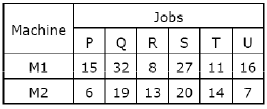The optimal make-span (in hours) of the shop is:

Solution: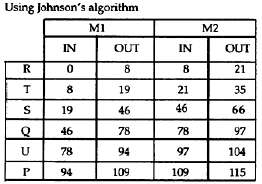QUESTION: 5

When using a simple moving average to forecast demand, one would

Solution:
QUESTION: 6

Four jobs are to be processed on a machine as per data listed in the table.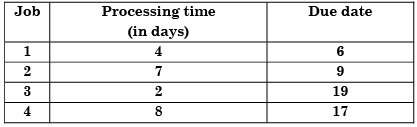If the Earliest Due-date (EDD) rule is used to sequence the jobs, the number of jobs delayed is:

Solution:

Alternating According to EDD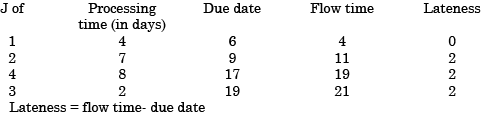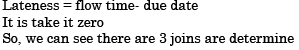QUESTION: 7

Four jobs are to be processed on a machine as per data listed in the table.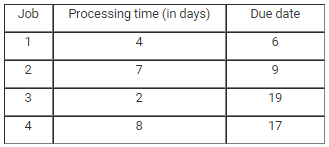Using the Shortest Processing Time (SPT) rule, total tardiness is

Solution: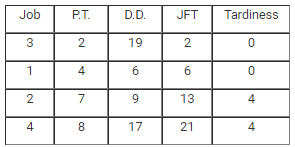Since, negative tardiness is not considered as delay i.e. considered as 0 tardiness.

So, Total tardiness =  0 + 0 + 4 + 4 = 8

QUESTION: 8

Which of the following forecasting methods takes a fraction of forecast error into account for the next period forecast?

Solution:
QUESTION: 9

Routing in production planning and control refers to the

Solution:
QUESTION: 10

The demand and forecast for February are 12000 and 10275, respectively. Using single exponential smoothening method (smoothening coefficient = 0.25), forecast for the month of March is:

Solution: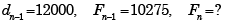According to single exponential smoothing method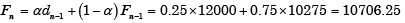QUESTION: 11

The routing function in a production system design is concerned with.

Solution:
QUESTION: 12

The sales of a product during the last four years were 860, 880, 870 and 890 units. The forecast for the fourth year was 876 units. If the forecast for the fifth year, using simple exponential smoothing, is equal to the forecast using a three period moving average, the value of the exponential smoothing constant a is:

Solution: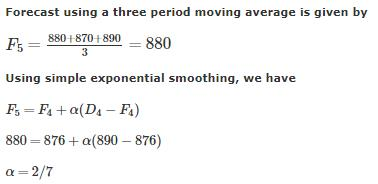.

QUESTION: 13

Consider the following statements:

Scheduling

1. Is a general timetable of manufacturing
2. Is the time phase of loading
3. Is loading all the work in process on
4. Machines according to their capacity

Which of the statements given above are correct?

Solution:
QUESTION: 14

For a product, the forecast and the actual sales for December 2002 were 25 and 20 respectively. If the exponential smoothing constant (α) is taken as 0.2, then forecast sales for January, 2003 would be:

Solution:

Use new forecast = old forecast + α (actual demand – old forecast)

QUESTION: 15

Consider the following statements:

1. Preparation of master production schedule is an iterative process
2. Schedule charts are made with respect to jobs while load charts are made with respect to machines
3. MRP is done before master production scheduling

Which of the statements given above are correct?

Solution:
QUESTION: 16

The sales of cycles in a shop in four consecutive months are given as 70, 68, 82, and 95. Exponentially smoothing average method with a smoothing factor of 0.4 is used in forecasting. The expected number of sales in the next month is:

Solution:

Let expected number of sales in the next month = ut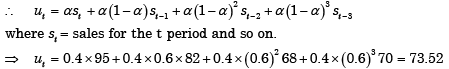QUESTION: 17

Which of the following factors are to be considered for production scheduling?

1. Sales forecast
2. Component design
3. Route sheet
4. Time stand ards

Select the correct answer using the codes given below:

Solution:
QUESTION: 18

In a forecasting model, at the end of period 13, the forecasted value for period 14 is 75. Actual value in the periods 14 to 16 are constant at 100. If the assumed simple exponential smoothing parameter is 0.5, then the MSE at the end of period 16 is:

Solution: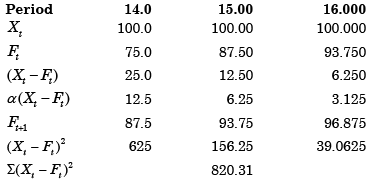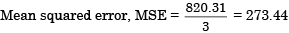QUESTION: 19

Assertion (A): Planning and scheduling of job order manufacturing differ from planning and scheduling of mass production manufacturing.

Reason (R): In mass production manufacturing, a large variety of products are manufactured in large quantity.

Solution:
QUESTION: 20

The most commonly used criteria for measuring forecast error is:

Solution: